Definitions of Square Dance Calls and Concepts
Exchange The Triangle [C3A]

From Twin Triangles. EN: 10

Do a total of three Triangle Circulates except that when you become 1) the Apex dancer (for Apex-to-Apex Triangles), or 2) the In-Facing Base dancer (for Base-to-Base Triangles); do your next Circulate to the other Triangle. (From Apex-to-Apex Triangles, the Circulate is an Interlocked Triangle Circulate; from Base-to-Base Triangles, the Circulate is to the diagonal opposite position.) Exchange The Triangle can be fractionalized into thirds. EN: 20

From Apex-to-Apex Wave-Based Triangles (Very Centers lead) EN: 30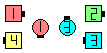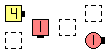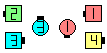beforeExchange The Triangles the left Triangle after 1/3... EN: 40 after
From Base-to-Base Tandem-Based Triangles (Trailing Centers lead) EN: 50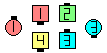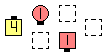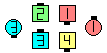beforeExchange The Triangles the left Triangle after 1/3... EN: 60 after

Note: As with all Exchanges, do Circulates staying on the inside of your formation until the crossover point, and then do Circulates staying on the outside of the other formation (and adjust to end on footprints of the original formation). EN: 70

Exchange The 3 By 1 Triangle [NOL]
(do a total of 4 Circulates). EN: 85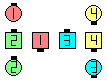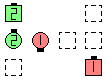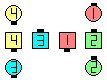beforeExchange The 3 By 1 Triangles the left Triangle after 1/4... EN: 90 after
Exchange The 3 By 1 Triangles [NOL]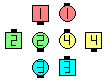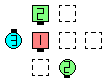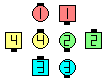beforeExchange The 3 By 1 Triangles the left Triangle after 1/4... EN: 110 after